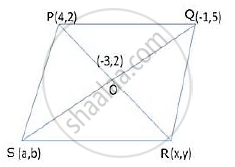# P (4, 2) and Q (-1, 5) are the vertices of parallelogram PQRS and (-3, 2) are the co-ordinates of the point of intersection of its diagonals. Find co-ordinates of R and S. - Mathematics

Sum

P (4, 2) and Q (-1, 5) are the vertices of parallelogram PQRS and (-3, 2) are the co-ordinates of the point of intersection of its diagonals. Find co-ordinates of R and S.

#### SolutionLet the coordinates of R and S be (x, y) and (a, b) respectively.
Mid-point of PR is O.

therefore O(-3,2)=O((4+x)/2,(2+y)/2)

-3=(4+x)/2, 2=(2+y)/2

-6=4+x, 4=2+y

x=-10, y=2

Hence, R = (−10,2)
Similarly, the mid-point of SQ is O.

therefore O(-3,2)=O((a-1)/2,(b+5)/2)

-3=(a-1)/2, 2=(b+5)/2

-6=a-1, 4=b+5

a=-5, b=-1

Hence, S=(-5,-1)

Thus, the coordinates of the point R and S are (-10, 2) and (-5, -1).

Concept: The Mid-point of a Line Segment (Mid-point Formula)
Is there an error in this question or solution?

#### APPEARS IN

Selina Concise Maths Class 10 ICSE
Chapter 13 Section and Mid-Point Formula
Exercise 13 (B) | Q 11 | Page 182